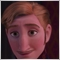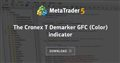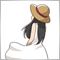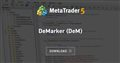mql4 formula not working in mql545

I am trying to convert the DeMarker mql4 indicator into to mql5.

https://www.mql5.com/en/code/8682

It is almost working, i am just having problems converting a formula which keeps resulting in inf (negative infinity) but works in mql4

Part of mql4 init()

//---- variable reset
e1=0; e2=0; e3=0; e4=0; e5=0; e6=0;
c1=0; c2=0; c3=0; c4=0;
n=0;
w1=0; w2=0;
b2=0; b3=0;
//
b2=Curvature*Curvature;
b3=b2*Curvature;
c1=-b3;
c2=(3*(b2+b3));
c3=-3*(2*b2+Curvature+b3);
c4=(1+3*Curvature+b3+3*b2);
n=DeMarker;
//
if (n<1) n=1;
n=1 + 0.5*(n-1);
w1=2/(n + 1);
w2=1 - w1;

mql4 start()

//---- DeMarker counted in the 1-st buffer

for(int i=limit; i>=0; i--)
{
DeMarkerBuffer[i]=(
iDeMarker(NULL,0,DeMarker+DeMStep*0,i)+
iDeMarker(NULL,0,DeMarker+DeMStep*1,i)+
iDeMarker(NULL,0,DeMarker+DeMStep*2,i)+
iDeMarker(NULL,0,DeMarker+DeMStep*3,i))*100/4-50;

e1=w1*DeMarkerBuffer[i] + w2*e1;
e2=w1*e1 + w2*e2;
e3=w1*e2 + w2*e3;
e4=w1*e3 + w2*e4;
e5=w1*e4 + w2*e5;
e6=w1*e5 + w2*e6;
//
DeMarkerTBuffer[i]=c1*e6 + c2*e5 + c3*e4 + c4*e3;
}

I cannot use iDeMarker indicator as you do in mql4 so my mql5 version is:

init()

//---- variable reset
e1=0; e2=0; e3=0; e4=0; e5=0; e6=0;
c1=0; c2=0; c3=0; c4=0;
n=0;
w1=0; w2=0;
b2=0; b3=0;
//
b2=Curvature*Curvature;
b3=b2*Curvature;
c1=-b3;
c2=(3*(b2+b3));
c3=-3*(2*b2+Curvature+b3);
c4=(1+3*Curvature+b3+3*b2);
n=DeMarker;
//
if (n<1) n=1;
n=1 + 0.5*(n-1);
w1=2/(n + 1);
w2=1 - w1;

DeMarker_handle1=iDeMarker(NULL,0,DeMarker);
DeMarker_handle2=iDeMarker(NULL,0,DeMarker+DeMStep*1);
DeMarker_handle3=iDeMarker(NULL,0,DeMarker+DeMStep*2);
DeMarker_handle4=iDeMarker(NULL,0,DeMarker+DeMStep*3);

if(DeMarker_handle1==INVALID_HANDLE || DeMarker_handle2==INVALID_HANDLE ||
DeMarker_handle3==INVALID_HANDLE || DeMarker_handle4==INVALID_HANDLE) {
IndicatorRelease(DeMarker_handle1);
IndicatorRelease(DeMarker_handle2);
IndicatorRelease(DeMarker_handle3);
IndicatorRelease(DeMarker_handle4);
return(INIT_FAILED);
}

my onCalculate()

for(int i=limit; i<rates_total && !_StopFlag; i++)
{
int MCopied1=CopyBuffer(DeMarker_handle1,0,BarsCalculated(DeMarker_handle1)-i,1,DeMarkerVal1);
int MCopied2=CopyBuffer(DeMarker_handle2,0,BarsCalculated(DeMarker_handle2)-i,1,DeMarkerVal2);
int MCopied3=CopyBuffer(DeMarker_handle3,0,BarsCalculated(DeMarker_handle3)-i,1,DeMarkerVal3);
int MCopied4=CopyBuffer(DeMarker_handle4,0,BarsCalculated(DeMarker_handle4)-i,1,DeMarkerVal4);

if (MCopied1!=1 || MCopied2!=1 || MCopied3!=1 || MCopied4!=1 ) return (rates_total);

double DeMarker1 = DeMarkerVal1;
double DeMarker2 = DeMarkerVal2;
double DeMarker3 = DeMarkerVal3;
double DeMarker4 = DeMarkerVal4;

DeMarkerBuffer[i]= (DeMarker1 + DeMarker2 + DeMarker3 + DeMarker4)*100/4-50;

e1=w1*DeMarkerBuffer[i] + w2*e1;
e2=w1*e1 + w2*e2;
e3=w1*e2 + w2*e3;
e4=w1*e3 + w2*e4;
e5=w1*e4 + w2*e5;
e6=w1*e5 + w2*e6;
//
DeMarkerTBuffer[i]=c1*e6 + c2*e5 + c3*e4 + c4*e3;
}

This is where I have the problem, the DeMarker indicator plots ok, smoother than the standard mql5 one, but e1 always results in inf, but obviously works in mql4, so my arrows, histogram, and other DeMarker line fail to plot correctly.The Cronex T Demarker GFC (Color) indicator
• www.mql5.com
Modification of the DeMarker indicator with additional color indication based on the histogram914

int MCopied1 = CopyBuffer(DeMarker_handle1, 0, BarsCalculated(DeMarker_handle1) - i - 1, 1, DeMarkerVal1);

If you don't add -1, it will be off by one bar.

You can simplify this by setting 'ArraySetAsSeries' to 'true'.

SetIndexBuffer(0, DeMarkerBuffer, INDICATOR_DATA);
ArraySetAsSeries(DeMarkerBuffer, true);
.
.
.
for (i = limit; i >= 0 && !IsStopped(); i--)
{
int MCopied1 = CopyBuffer(DeMarker_handle1, 0, i, 1, DeMarkerVal1);

The 'limit' is always fixed because it's using T3 calculations. (See MT4 file) .45

If you don't add -1, it will be off by one bar.

You can simplify this by setting 'ArraySetAsSeries' to 'true'.

The 'limit' is always fixed because it's using T3 calculations. (See MT4 file) .

Thanks I noticed the (I) was wrong after I worked out how to debug the indicator!

I can more easily see the variable outputs.

I am still getting weird results, but will come back if I get stuck.45

I finally got it working.

for(int i=limit; i<rates_total && !_StopFlag; i++)
{
if (i < DeMarker+DeMStep*3) continue;

int MCopied1=CopyBuffer(DeMarker_handle1,0,BarsCalculated(DeMarker_handle1)-i,1,DeMarkerVal1);
int MCopied2=CopyBuffer(DeMarker_handle2,0,BarsCalculated(DeMarker_handle2)-i,1,DeMarkerVal2);
int MCopied3=CopyBuffer(DeMarker_handle3,0,BarsCalculated(DeMarker_handle3)-i,1,DeMarkerVal3);
int MCopied4=CopyBuffer(DeMarker_handle4,0,BarsCalculated(DeMarker_handle4)-i,1,DeMarkerVal4);

if (MCopied1!=1 || MCopied2!=1 || MCopied3!=1 || MCopied4!=1 ) continue;

//      double DeMarker1 = (double) DoubleToString(DeMarkerVal1,5);
double DeMarker1 = DeMarkerVal1;
double DeMarker2 = DeMarkerVal2;
double DeMarker3 = DeMarkerVal3;
double DeMarker4 = DeMarkerVal4;

DeMarkerBuffer[i]= (DeMarker1 + DeMarker2 + DeMarker3 + DeMarker4)*100/4-50;

e1=w1*DeMarkerBuffer[i] + w2*e1;
e2=w1*e1 + w2*e2;
e3=w1*e2 + w2*e3;
e4=w1*e3 + w2*e4;
e5=w1*e4 + w2*e5;
e6=w1*e5 + w2*e6;

DeMarkerTBuffer[i]=c1*e6 + c2*e5 + c3*e4 + c4*e3;
}